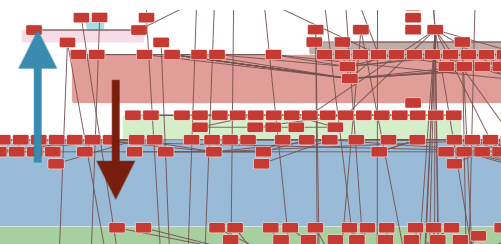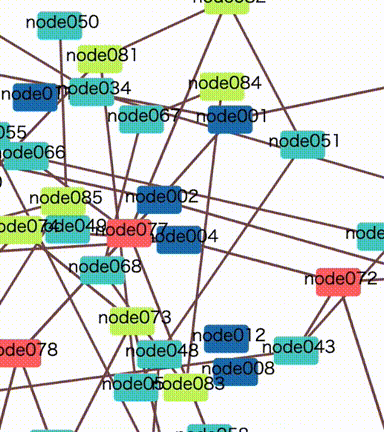# inet-henge for large scale network diagrams

inet-henge is a d3.js based network diagram generator that calculates node positions automatically. The input is a simple json like below, it requires no position information such as x-y coordinates.

```{
"nodes": [
{ "name": "A" },
{ "name": "B" }
],

{ "source": "A", "target": "B" }
]
}
```

The auto-layout works good and fast enough for small networks but it gets more complex and painfully slow when you have many nodes and links in a diagram. It'll take a couple of minutes to calculate the position of 300+ nodes and 1400 links for instance.

As a performance improvement, I've introduced a new option to tweak the auto-layout algorithm, especially for large scale network diagrams. When I tried my test data comprised of 800 nodes and 950 links, it only took <20 secs to determine node positions. I know it's still slow but acceptable hopefully, as it should be a one-shot calculation - inet-henge can cache the result for further rendering.

### So why was it so slow?

Besides inet-henge calculates the node positions repeatedly in each time slice just like force layout of d3.js, extra constraints are added to iteration:

1. Group nodes with bounding boxes
2. Prevent nodes and groups from overlapping with each other

If you have huge groups having many nodes, it sometimes wastes ticks to pass by each other. In an example diagram below, it looks hard for a blue group to pass through a red one. It might get stuck for many ticks or never pass in the worst case.The same thing will happen even in a no group diagram. Every node collides with each other many times and it prevents position calculation from fast convergence.### The new option `initialTicks`

For such a large scale network diagram, you can specify the number of initial "unconstrained" ticks.

```const diagram = new Diagram('#diagram', 'data.json', {initialTicks: 100, ticks: 100});
```

With this option, inet-henge calculates the layout in two iteration phases:

1. Initial iteration with no constraints. ( default: 0 tick )
• Every group or node can transparently pass through each other in this phase.
2. The main iteration with constraints that apply groups as bounding boxes, prevent nodes and groups from overlapping with each other, and so on. ( default: 1000 ticks )

Note: If you increase `initialTicks`, inet-henge calculates faster in exchange for network diagram precision so that you can decrease `ticks` which is the number of main iteration steps.

20 ~ 100 `initialTicks` and 70 ~ 100 `ticks` should be good start for 800 nodes with 950 links for example. It takes 20 seconds to render in my benchmark environment.

The `initialTicks` option is very new and not tested enough due to a lack of actual network topology data. If you're interested in this project and drawing your network diagram, please reach out to me ( Twitter ) with your use case. Any feedback will be appreciated.